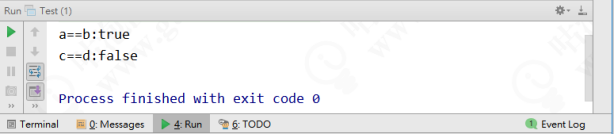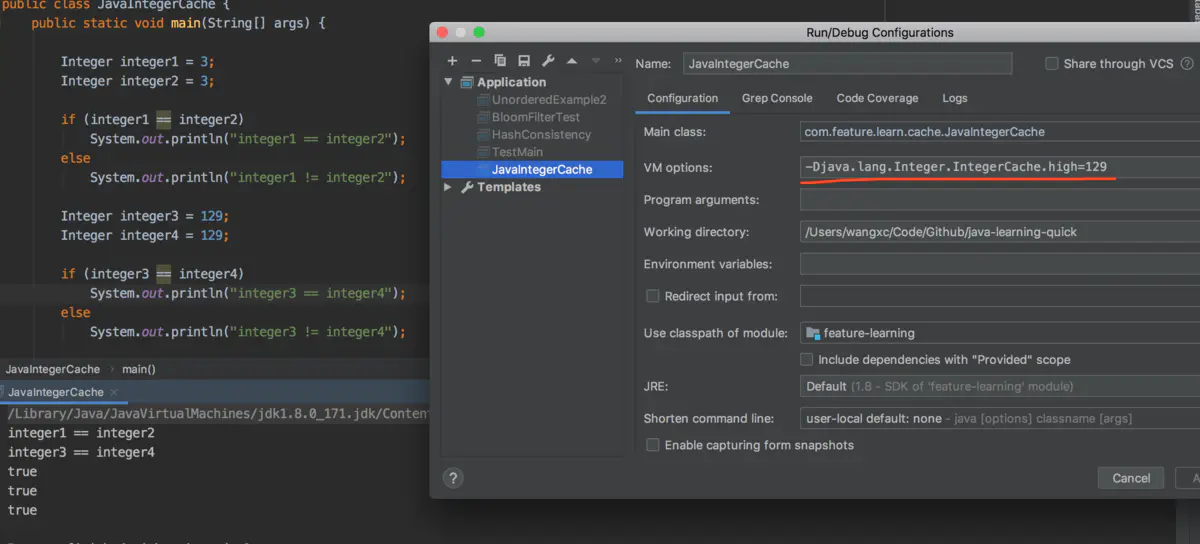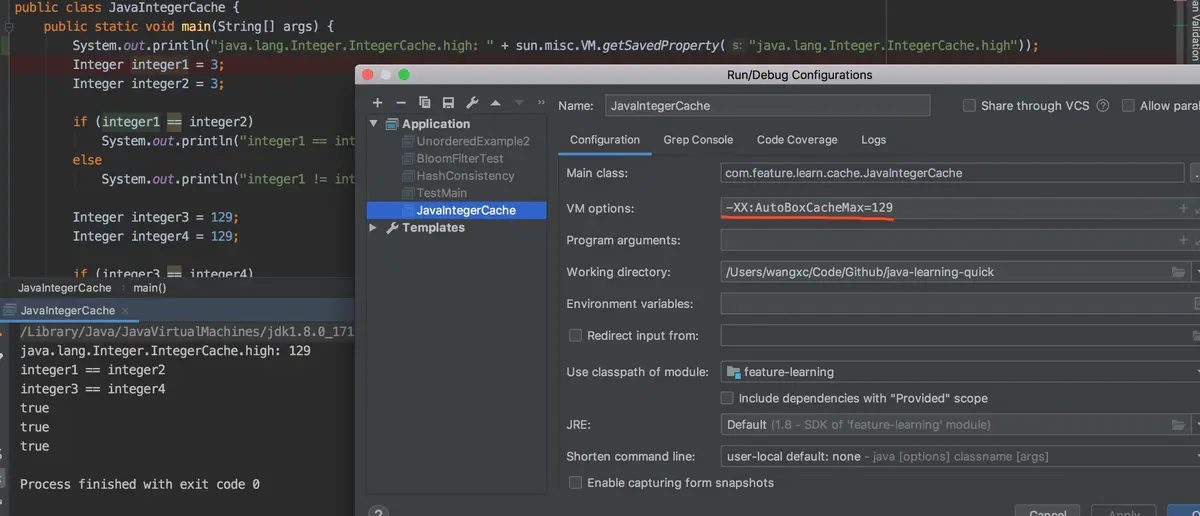# 就因為把int改成Integer，第2天被辭了

## 1 故事背景

``````
public static void main(String[] args) {

Integer a = Integer.valueOf(100);
Integer b = 100;

Integer c = Integer.valueOf(129);
Integer d = 129;

System.out.println("a==b:" + (a==b));
System.out.println("c==d:" + (c==d));
}

````````````
public final class Integer extends Number implements Comparable<Integer> {

...

public static Integer valueOf(int i) {

if (i >= IntegerCache.low && i <= IntegerCache.high)
return IntegerCache.cache[i + (-IntegerCache.low)];
return new Integer(i);

}

...

}

``````

``````
private static class IntegerCache {
// 最小值
static final int low = -128;
// 最大值，支援自定義
static final int high;
// 快取陣列
static final Integer cache[];

static {
// 最大值可以通過屬性配置來改變
int h = 127;
String integerCacheHighPropValue =
sun.misc.VM.getSavedProperty("java.lang.Integer.IntegerCache.high");
// 如果設定了對應的屬性，則使用該值
if (integerCacheHighPropValue != null) {
try {
int i = parseInt(integerCacheHighPropValue);
i = Math.max(i, 127);
// 最大陣列大小為Integer.MAX_VALUE
h = Math.min(i, Integer.MAX_VALUE - (-low) -1);
} catch( NumberFormatException nfe) {
// If the property cannot be parsed into an int, ignore it.
}
}
high = h;

cache = new Integer[(high - low) + 1];
int j = low;
// 將low-high範圍內的值全部例項化並存入陣列中當快取使用
for(int k = 0; k < cache.length; k++)
cache[k] = new Integer(j++);

// range [-128, 127] must be interned (JLS7 5.1.7)
assert IntegerCache.high >= 127;
}

private IntegerCache() {}
}

``````

Returns an Integer instance representing the specified int value. If a new Integer instance is not required, this method should generally be used in preference to the constructor Integer(int), as this method is likely to yield significantly better space and time performance by caching frequently requested values. This method will always cache values in the range -128 to 127, inclusive, and may cache other values outside of this range

128～127的資料在int範圍內是使用最頻繁的，為了減少頻繁建立物件帶來的記憶體消耗，這裡其實是用到了享元模式，以提高空間和時間效能。

JDK增加了這一預設的範圍並不是不可變，我們在使用前可以通過設定-Djava.lang.Integer.IntegerCache.high=xxx或者設定-XX:AutoBoxCacheMax=xxx來修改快取範圍，如下圖：Java Language Specification 的原文解釋如下：

Ideally, boxing a given primitive value p, would always yield an identical reference. In practice, this may not be feasible using existing implementation techniques. The rules above are a pragmatic compromise. The final clause above requires that certain common values always be boxed into indistinguishable objects. The implementation may cache these, lazily or eagerly. For other values, this formulation disallows any assumptions about the identity of the boxed values on the programmer's part. This would allow (but not require) sharing of some or all of these references. This ensures that in most common cases, the behavior will be the desired one, without imposing an undue performance penalty, especially on small devices. Less memory-limited implementations might, for example, cache all char and short values, as well as int and long values in the range of -32K to +32K.

## 2 關於Integer和int的比較

1. 由於Integer變數實際上是對一個Integer物件的引用，所以兩個通過new生成的Integer變數永遠是不相等的（因為new生成的是兩個物件，其記憶體地址不同）。
``````
Integer i = new Integer(100);
Integer j = new Integer(100);
System.out.print(i == j); //false

``````
1. Integer變數和int變數比較時，只要兩個變數的值是向等的，則結果為true（因為包裝類Integer和基本資料型別int比較時，java會自動拆包裝為int，然後進行比較，實際上就變為兩個int變數的比較）
``````
Integer i = new Integer(100);
int j = 100；
System.out.print(i == j); //true

``````
1. 非new生成的Integer變數和new Integer()生成的變數比較時，結果為false。（因為 ①當變數值在-128 - 127之間時，非new生成的Integer變數指向的是java常量池中的物件，而new Integer()生成的變數指向堆中新建的物件，兩者在記憶體中的地址不同；②當變數值在-128 - 127之間時，非new生成Integer變數時，java API中最終會按照new Integer(i)進行處理（參考下面第4條），最終兩個Interger的地址同樣是不相同的）
``````
Integer i = new Integer(100);
Integer j = 100;
System.out.print(i == j); //false

``````
1. 對於兩個非new生成的Integer物件，進行比較時，如果兩個變數的值在區間-128到127之間，則比較結果為true，如果兩個變數的值不在此區間，則比較結果為false

## 3 擴充套件知識

boolean - - - Bloolean - -
char 6bit Unicode 0 Unic ode 2(16)-1 Character 0~127
byte 8bit -128 +127 Byte -128~127
short 16bit -2(15) 2(15)-1 Short -128~127
int 32bit -2(31) 2(31)-1 Integer -128~127 支援
long 64bit -2(63) 2(63)-1 Long -128~127
float 32bit IEEE754 IEEE754 Float -
double 64bit IEEE754 IEEE754 Double -
void - - - Void - -

## 4 使用享元模式實現資料庫連線池

``````
public class ConnectionPool {

private Vector<Connection> pool;

private String url = "jdbc:mysql://localhost:3306/test";
private String username = "root";
private String password = "root";
private String driverClassName = "com.mysql.jdbc.Driver";
private int poolSize = 100;

public ConnectionPool() {

pool = new Vector<Connection>(poolSize);

try{
Class.forName(driverClassName);
for (int i = 0; i < poolSize; i++) {
}
}catch (Exception e){
e.printStackTrace();
}

}

public synchronized Connection getConnection(){
if(pool.size() > 0){
Connection conn = pool.get(0);
pool.remove(conn);
return conn;
}
return null;
}

public synchronized void release(Connection conn){